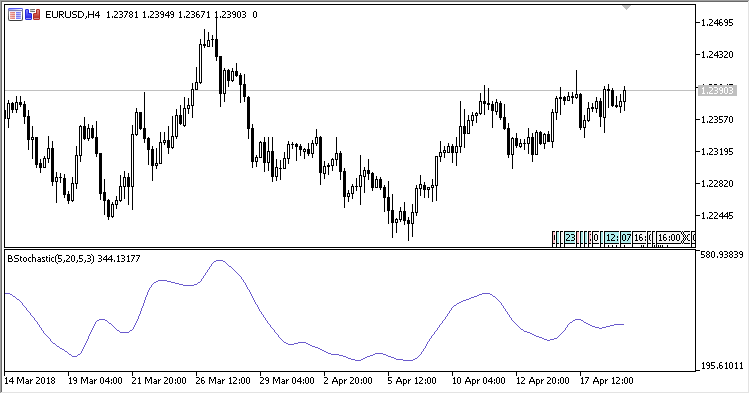# Blau_TStoch – indicator for MetaTrader 5

• A+
category：MT5 INDICATORS

The William Blau Stochastic indicator shows the william Blau stochastic.

The indicator has five input parameters:

• Period - calculation period;
• First smooth period - the initial smoothing period;
• Second smooth period - the secondary smoothing period;
• Third smooth period - the resulting smoothing period;
• Applied price - price used for calculations.

Calculations:

```Blau_Stochastic = EMA(EMA(EMA(Stoch, First smooth period), Second smooth period), Third smooth period), where
Stoch[i] = Price[i]-Min,
Min is the lowest price within the range from (i-Period+1) to (i)
```